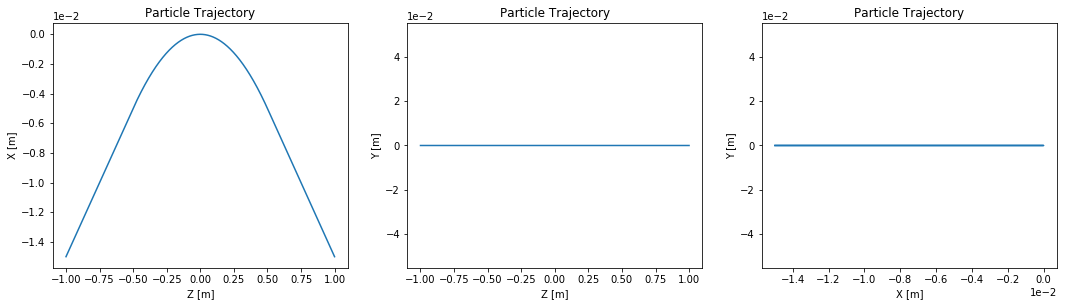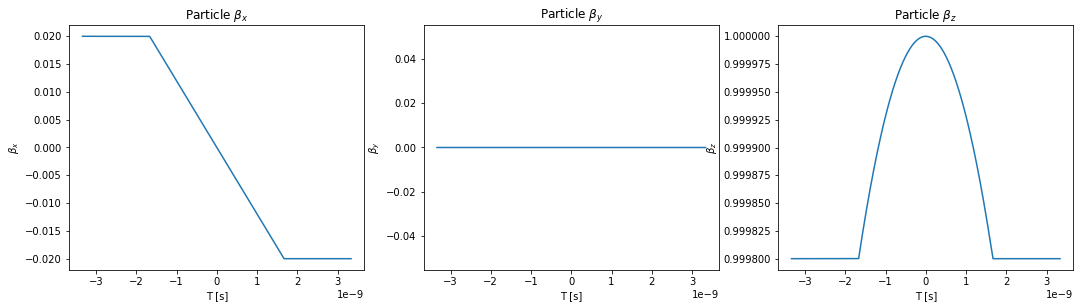# Example 001: Trajectory in a Dipole¶

In this example the electron trajectory in a dipole is calculated and plotted. Some attempt is made to explain each step along the way.

We will first start by looking at the trajectory where we set the initial conditions for a particle outside of the dipole magnet (as is typical in the case of looking at undulators).

In :
# This has nothing to do with OSCARS, but it puts the matplotlib plots inline in the notebook
%matplotlib inline

# Import the OSCARS SR module
import oscars.sr

# Import basic plot utilities.  You don't need these to run OSCARS, but it's used here for basic plots
from oscars.plots_mpl import *

OSCARS v2.1.8 - Open Source Code for Advanced Radiation Simulation
Brookhaven National Laboratory, Upton NY, USA
http://oscars.bnl.gov
oscars@bnl.gov

In :
# Create a new OSCARS object.  Default to 8 threads and always use the GPU if available


## Create the dipole field¶

Here we create the dipole field. Here bfield represents magnetic field [$B_x, B_y, B_z$]. The width is also in vector form which allows you to specify the spatial extent of the uniform field. If one component is zero then that spatial dimension is ignored (the field extends to $\pm \infty$ in that dimension).

Typically clear_magnetic_fields() is called before adding a field in notebooks only to save time when making changes and rerunning sections of the code so it is not strictly necessary.

In :
# Clear any existing fields (just good habit in notebook style) and add an undulator field
osr.clear_bfields()
osr.add_bfield_uniform(bfield=[0, -0.4, 0], width=[0, 0, 1])

In :
# Just to check the field that we added seems visually correct
plot_bfield(osr)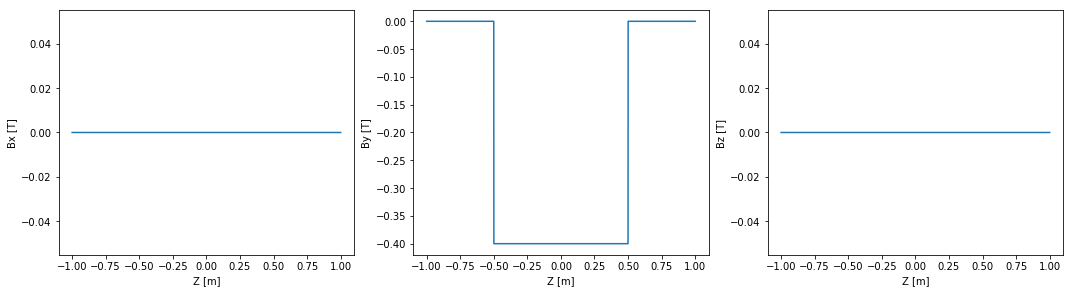Now we add a simple particle beam. There are a few important things to understand about the setup of magnetic fields and particle beams, namely positions and times. You can define the initial position and time of a particle beam to be anywhere you like. The default initial time for a beam is t=0. The default initial direction is d0=[0, 0, 1]. The default initial position is x0=[0, 0, 0], but in some cases it makes more sense to specify this before the field of interest, in this case at z=-1 as in the first example([0, 0, -1]).

One MUST specify ctstartstop. This is the start and stop time of the calculation. In this example we will start the calculation at t=0 and go to t=2 (given in units of ct) since the beam is relativistic. In this example you can specify the start time as less than 0 which is useful if you want to propogate the particle backwars in time. This is useful for instance if you have a bending magnet before the undulator that you wish to include.

clear_particle_beams() is called, again for convenience, but it is not necessary.

In set_particle_beam() the default particle type is 'electron', but other are possible (see documentation)

In :
# Setup beam similar to NSLSII
osr.clear_particle_beams()
osr.set_particle_beam(x0=[0, 0, -1], energy_GeV=3, current=0.500)

# Set the start and stop times for the calculation
osr.set_ctstartstop(0, 2)

In :
# Verify input information - print all to screen
osr.print_all()

TParticleBeamContainer has 1 beams
Name:             _beam0
Weight:           1
X0:               (0, 0, -1)
U0:               (0, 0, 1)
T0:               0 [m]  0 [s]
E0:               3
SigmaE:           6.95268e-310
Current           0.5
Emittance         (6.93657e-310, 6.93664e-310)
V-direction       (-0, 1, -0)
H-direction       (1, -0, -0)
BeamDistribution  filament
TwissBeta         (6.93663e-310, 4.94066e-324)
TwissAlpha        (6.93663e-310, 6.93663e-310)
TwissGamma        (6.95268e-310, 4.94066e-324)
Twiss Lattice Ref (0, 0, 0)
TwissBetaX0       (2.77626e-309, 1.38733e-309)
TwissAlphaX0      (1.38893e-309, 6.93663e-310)
TwissGammaX0      (6.95268e-310, 4.94066e-324)
Eta               (0, 0)

*Magnetic Fields*
TFieldContainer has 1 fields
TField3D_UniformBox
Name
Field               (0, -0.4, 0)
Width               (0, 0, 1)
Rotations           (0, 0, 0)
Center              (0, 0, 0)
Frequency           0
FrequencyPhase      0
TimeOffset          0

*Electric Fields*
TFieldContainer has 0 fields

*Drift Volumes*
TDriftVolumeContainer has 0 DriftVolumes

*GPUs*
Use GPU Globally: 1
Number of GPUs: 1

GPU 0
Memory Clock Rate (KHz): 2700000
Memory Bus Width (bits): 256
Peak Memory Bandwidth (GB/s): 172.800000

Number of Threads to use: 8

*Trajectory Calculation Globals*
Method:    RK4
Precision: 1e-06



## Calculate Trajectory¶

Now we calculate the trajectory and plot it. It is enough to call calculate_trajectory(). If you are doing other calculations (flux, spectra, power density) it is not necesary to call this since it is called internally.

In :
# Run the particle trajectory calculation
trajectory = osr.calculate_trajectory()

# Plot the trajectory position and velocity
plot_trajectory_position(trajectory)
plot_trajectory_velocity(trajectory)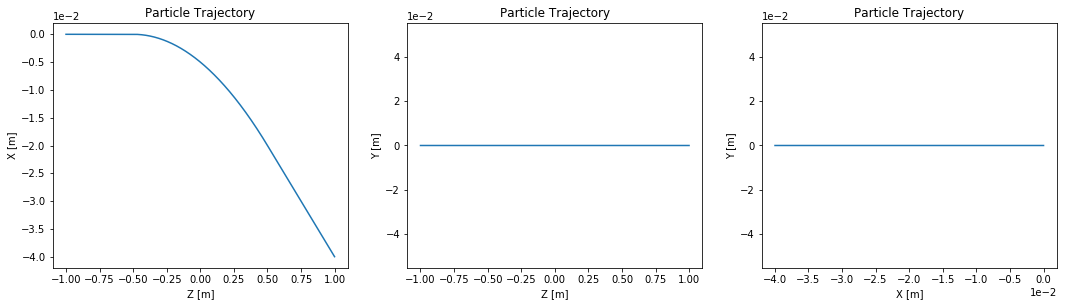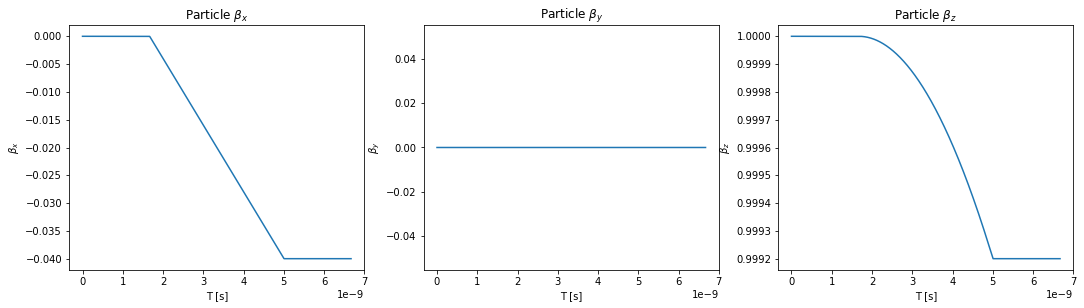## Alternate Starting Position (and backward propogation)¶

Here we set the beam t0 in the middle of the dipole. This is convienent for some calculations.

In order to propogate the particle backwards from the point given for its initial conditions one simply changes the definition of either the beam t0 or the ctstartstop. Here we will change ctstartstop and x0 parameters.

In :
# Setup beam similar to NSLSII
osr.clear_particle_beams()
osr.set_particle_beam(energy_GeV=3, current=0.500)

# Set the start and stop times for the calculation
osr.set_ctstartstop(-1, 1)

In :
# Run the particle trajectory calculation
trajectory = osr.calculate_trajectory()

# Plot the trajectory position and velocity
plot_trajectory_position(trajectory)
plot_trajectory_velocity(trajectory)